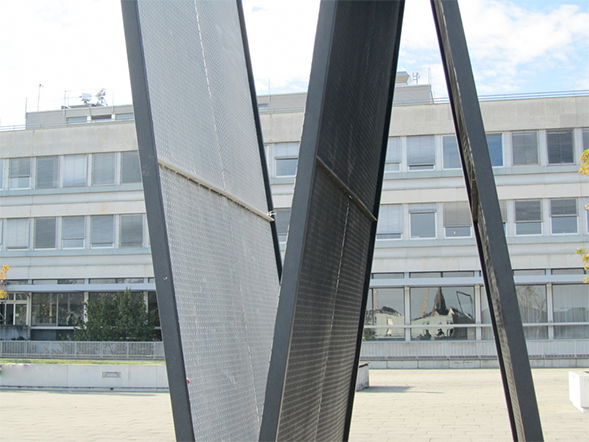## Mathematical Institute## Welcome to the website of the Mathematical Institute

The Mathematical Institute is responsible for Bachelor- and Master-level teaching for mathematics students, as well as for service courses in other areas of study. In addition the Institute undertakes research in such areas of mathematics as algebra, logic, analysis, numerical analysis, and geometry and topology. The Mathematical Institute is a part of the Department of Mathematics und Statistics.